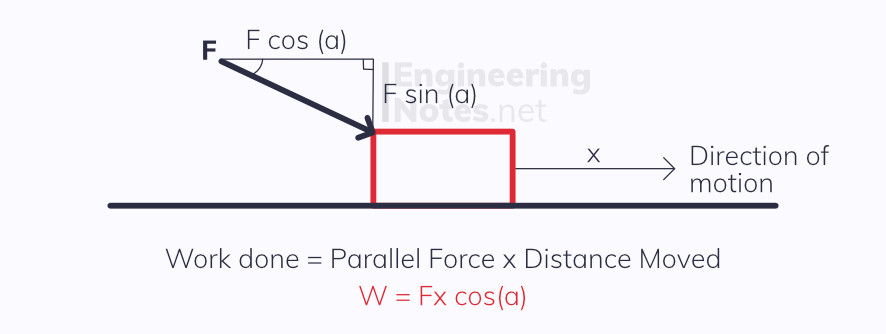#### Notes by Category University Engineering

Electronics*
Mathematics*
Mechanics & Stress Analysis*
Rate these notesNot a fanNot so goodGoodVery goodBrillRate these notes
• A-Level Physics

# Work, Energy & Power

Work done is the amount of energy transferred from one form to another when a force causes movement.

The units of work are Joules, J
W = Fx Work Done = Force × distance moved (in the same direction as the force)When the force is not in the same direction as the movement of the object, you need to work out the correct component force using trigonometry.

You must remember to do this!

## Energy

One of the fundamental laws of physics is the idea that energy cannot be created. This is known as the Principle of Conservation of Energy and states:

The total energy in a closed system always remains constant - energy can never be created or destroyed, it can only ever be transferred from one form to another.

### Some of these forms include:

• Kinetic

• Gravitational Potential

• Elastic Potential

• Electrical Potential

• Nuclear

• Internal (heat/thermal)

### Kinetic Energy

All moving objects have kinetic energy:

KE = 1/2 m v^2 Kinetic Energy = 1/2 x mass x velocity squared

This equation can be derived easily from work done and SUVAT: